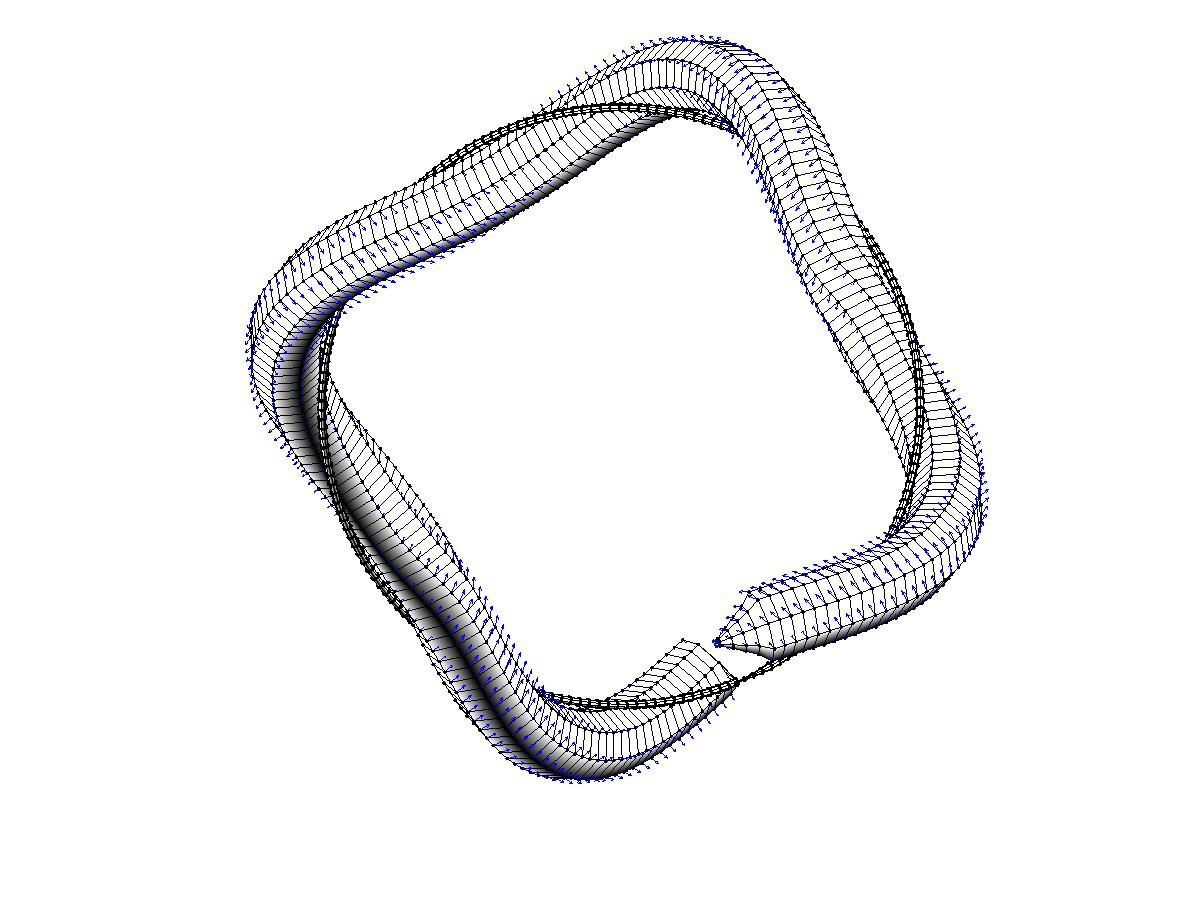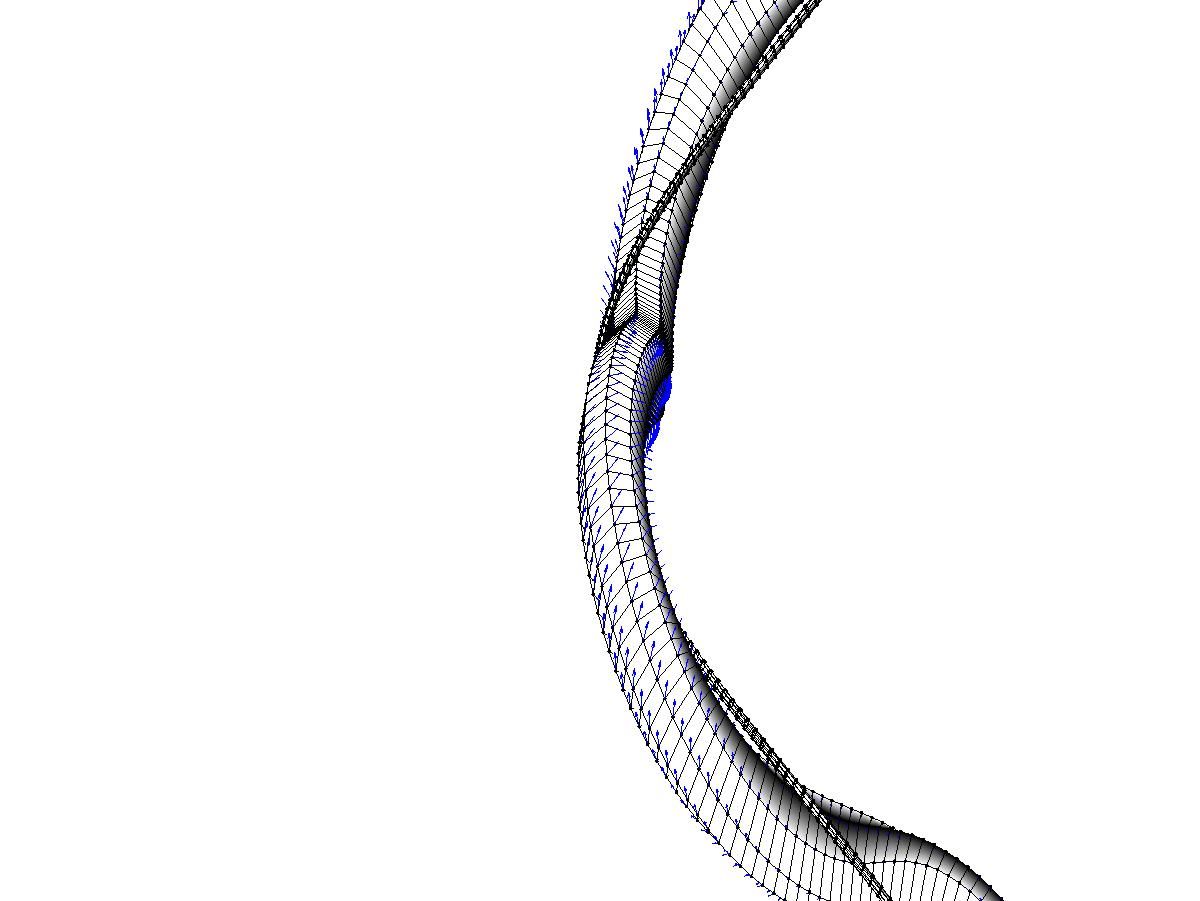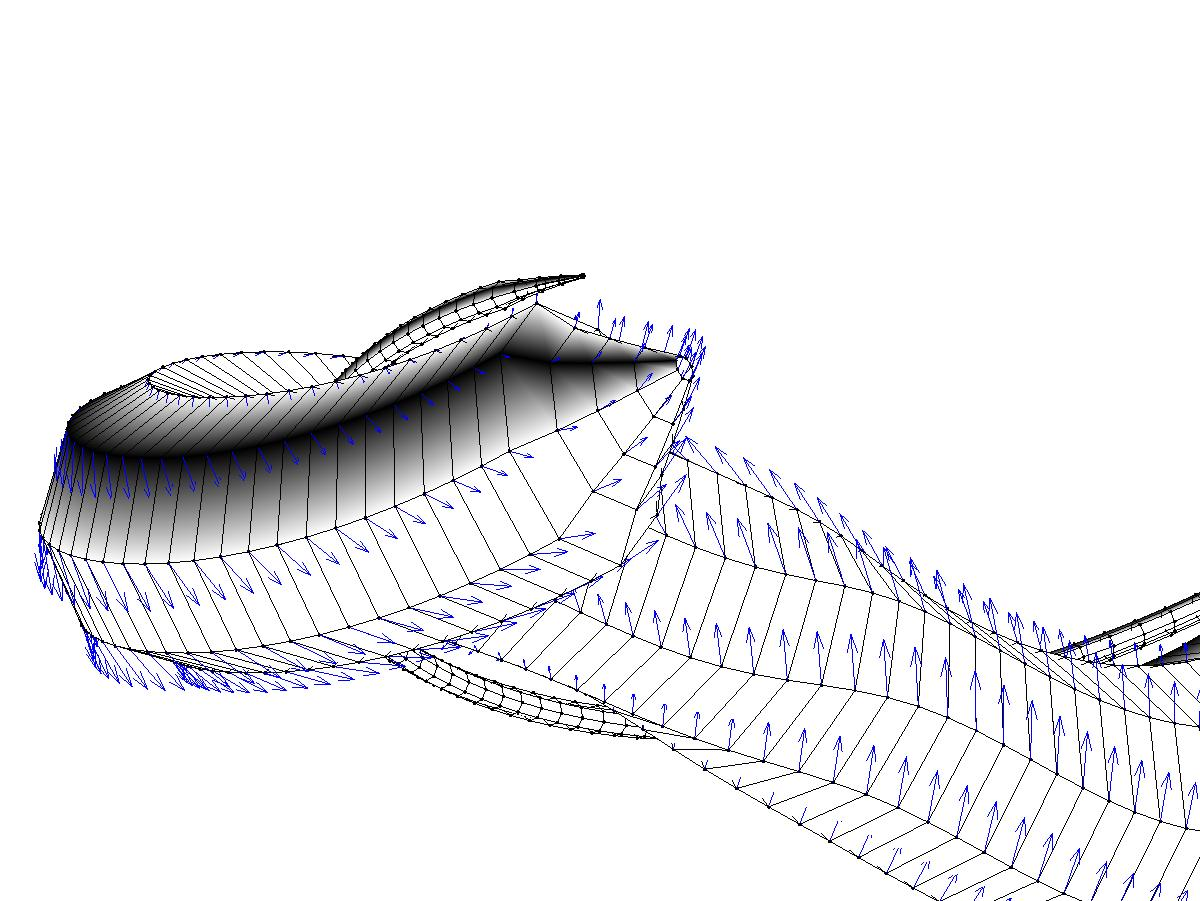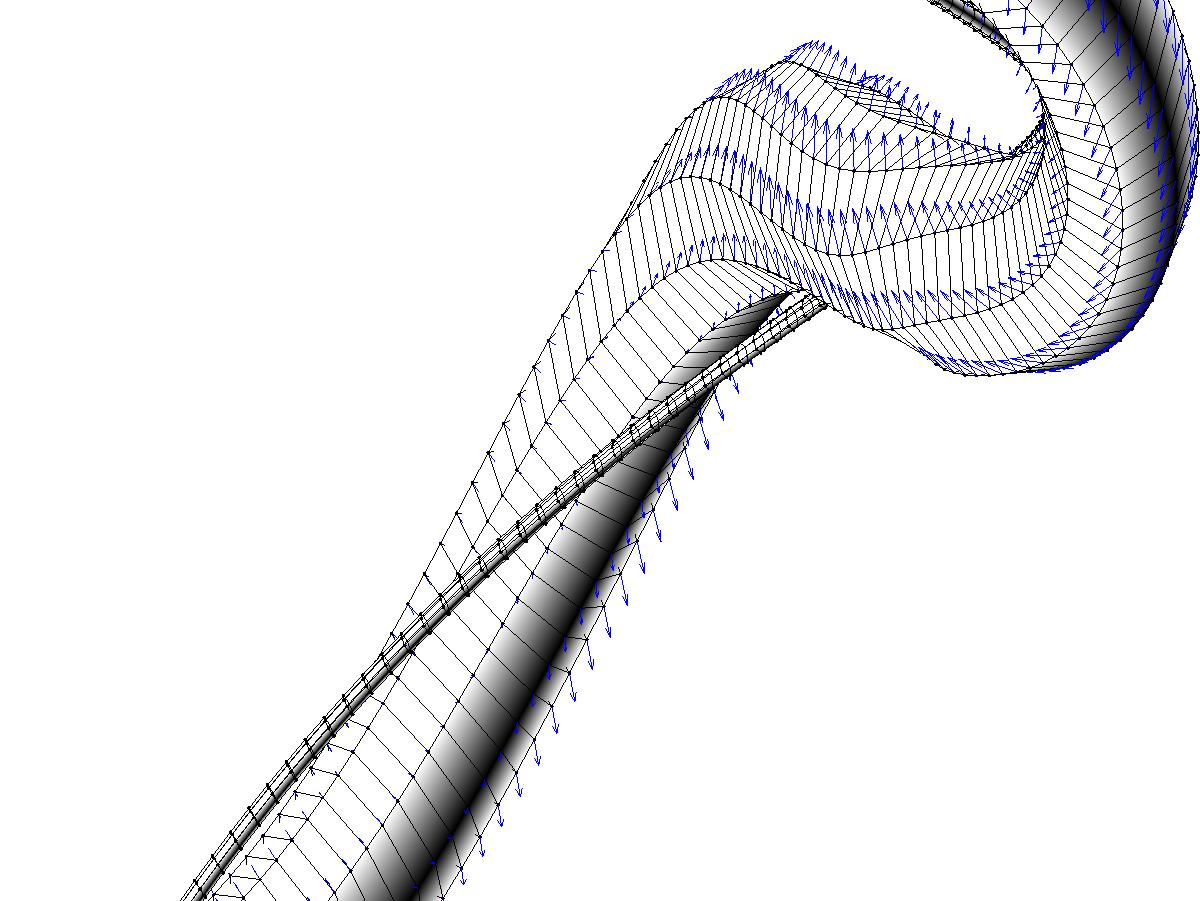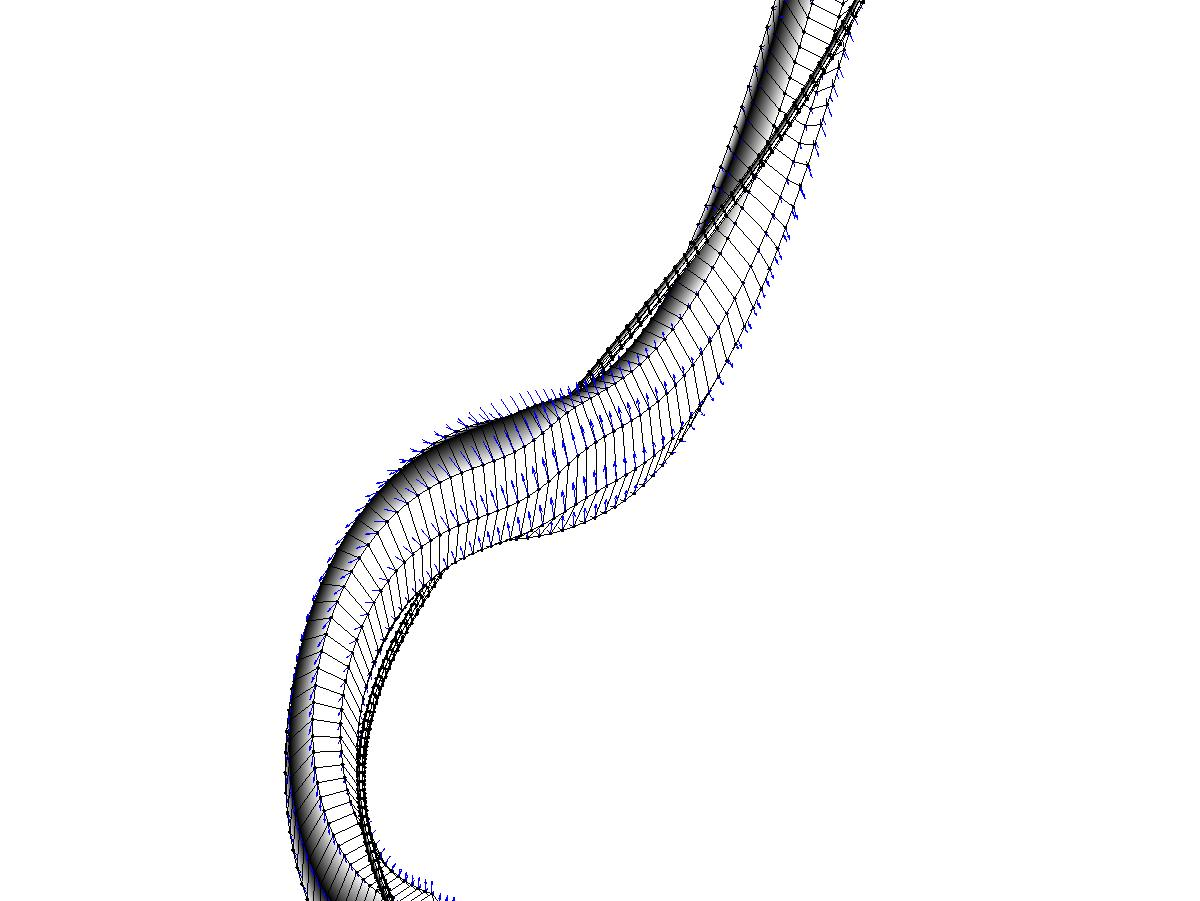Mathematical modeling of motility of microorganisms.

Homepage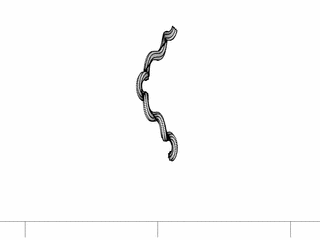Fluid dynamics usually described in terms of Navier-Stokes equations. But microorganisms are so small that we can approximate non-linear Navier-Stokes equations by linear Stokes equations. This simplification allows to use reciprocal theorem (similar to the theorem used for Laplace equations in order to derive solution in terms of Green functions) to derive solution of Stokes equations in terms of integral equations (nice review of these methods can be found in the book of Pozrikidis, C. 1992 Boundary Integral and Singularity Methods for Linearized Viscous Flow. Cambridge University Press.) In order to approximate integral equations we've used method of regularized stokeslets, to describe boundary forces we've used model of elastic spirochete's surface proposed by Lisa Fauci which is based on system of connected springs as well as "motor" springs to simulate motors of real spirochete. For calculation of resulting system of ordinary differential equations we've used high order explicit method for stiff differential equations. We've calculated motion of spirochete as an elastic body as well as global swimming characteristics (rotational and translational resistance matrixes and ratio of translational and rotational velocities) of the solid body of helical shape:
R. Cortez, L. Fauci, A. Medovikov 2003. The method of regularized stokeslets in three dimensions: analysis, validation, and application to helical swimming., Physics of Fluids 17, 031504 2005(has been selected for the March 1, Volume 9, Issue 5, 2005 of Virtual Journal of Biological Physics Research).